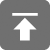# 工学1号馆

home

## HDOJ 1002 A + B Problem II

Wu Yudong    May 08, 2018     HDOJ   526

Problem – 1002

 Problem Description I have a very simple problem for you. Given two integers A and B, your job is to calculate the Sum of A + B. Input The first line of the input contains an integer T(1<=T<=20) which means the number of test cases. Then T lines follow, each line consists of two positive integers, A and B. Notice that the integers are very large, that means you should not process them by using 32-bit integer. You may assume the length of each integer will not exceed 1000. Output For each test case, you should output two lines. The first line is “Case #:”, # means the number of the test case. The second line is the an equation “A + B = Sum”, Sum means the result of A + B. Note there are some spaces int the equation. Output a blank line between two test cases. Sample Input 2 1 2 112233445566778899   998877665544332211 Sample Output Case 1: 1 + 2 = 3 Case 2: 112233445566778899 + 998877665544332211 = 1111111111111111110

#include<stdio.h>
#include<string.h>
#include<ctype.h>
#include<math.h>

char a,b;

void sum(char *s1, char *s2) //加法运算函数
{
int len1,len2,i,t,flag;
char *p,*q;
int c={0};
len1=strlen(s1); len2=strlen(s2);
//使用字符串存储加数与被加数
i=t=flag=0;
printf("%s + ",a); //控制输出语句
printf("%s = ",b);
p=strrev(s1); //转置字符串
q=strrev(s2);
while(*p || *q){
if(*p) t+=*p-'0';
if(*q) t+=*q-'0';
t+=flag;
if(t>=10){t=t%10; c[i++]=t; flag=1;}
else {c[i++]=t; flag=0;}
p++; q++;
t=0;
}
if(flag) c[i]+=1;
else i--;

for(; i>=0; i--){
printf("%d",c[i]); //输出结果
}
}

void solve()
{
int i,n,t;
i=1;
scanf("%d",&n);
t=n;
getchar();
while(n--){
scanf("%s",a);  scanf("%s",b);
printf("Case %d:\n",i);
sum(a,b);
if(i!=t) printf("\n\n");
else printf("\n");
i++;
memset(a,'\0',strlen(a)*sizeof(char)); //清空字符串
memset(b,'\0',strlen(b)*sizeof(char));
}
}

int main()
{
solve();
getchar();
getchar();
return 0;
}